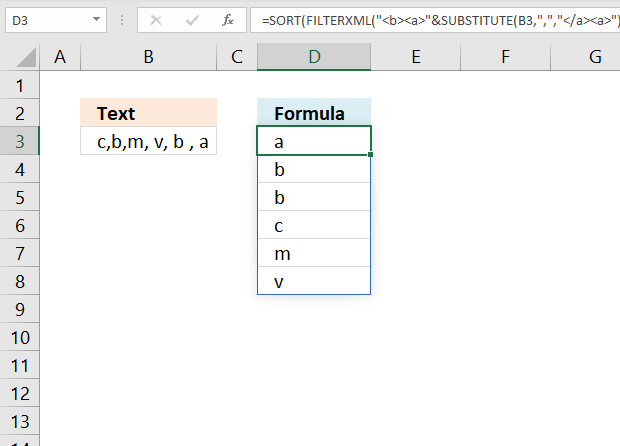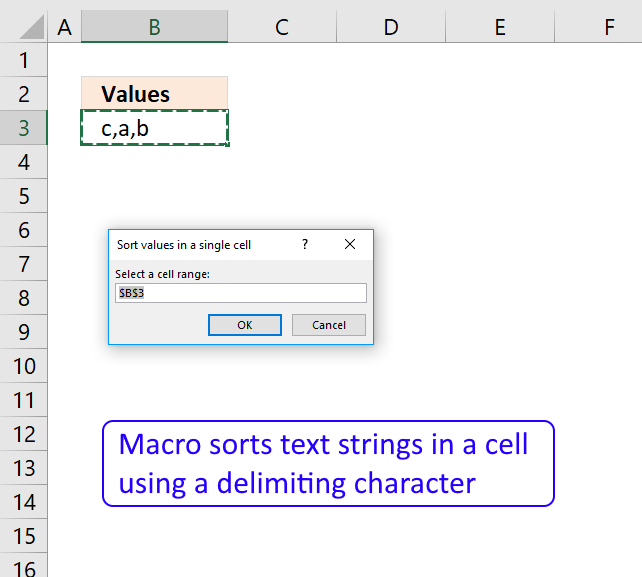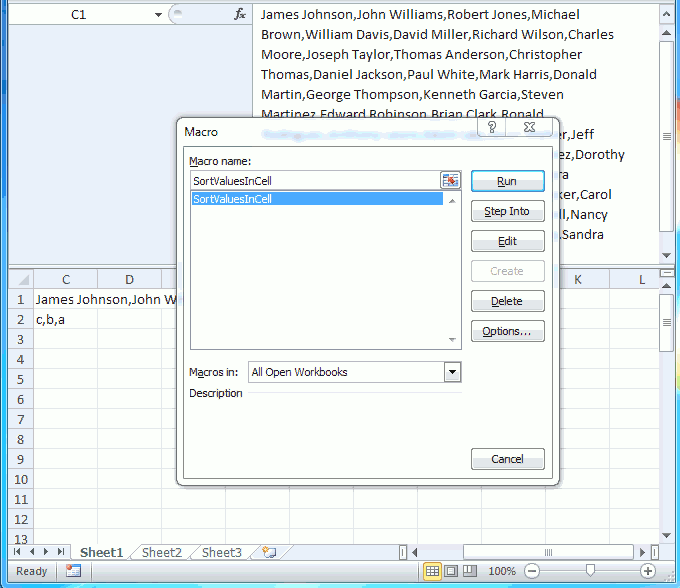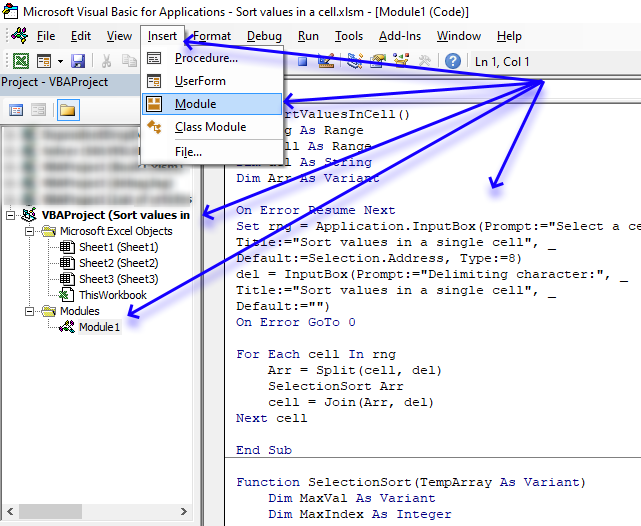Author: Oscar Cronquist Article last updated on July 06, 2021This article demonstrates a macro and a formula that allows you to sort delimited data in a cell or cell range from A to Z.  It sorts the values in a cell each not cells combined.

## 1 Sort values in a single cell based on a delimiting character (Formula)The image above shows a formula in cell D3 that sorts multiple values in cell B3 based on a delimiting character. The formula returns an array of values, Excel 365 users may enter the formula as a regular formula, however, previous versions must enter the formula as an array formula.

The FILTERXML function is an Excel 2013 function.

Formula in cell D3:

=SORT(FILTERXML("<b><a>"&SUBSTITUTE(B3, ",", "</a><a>")&"</a></b>","//a"))

### 1.1 Explaining formula in cell D3

#### Step 1 - Replace the delimiting character with a XML tag name

The SUBSTITUTE function substitutes a given string with another string, all found instances are replaced.

SUBSTITUTE(B3, ",", "</a><a>")

becomes

SUBSTITUTE("c,b,m, v, b , a",",","</a><a>")

and returns

"c</a><a>b</a><a>m</a><a> v</a><a> b </a><a> a"

#### Step 2 - Concatenate XML tags

The ampersand character concatenates strings in an excel formula.

"<b><a>"&SUBSTITUTE(B3, ",", "</a><a>")&"</a></b>"

becomes

"<b><a>"&"c</a><a>b</a><a>m</a><a> v</a><a> b </a><a> a"&"</a></b>"

and returns

"<b><a>c</a><a>b</a><a>m</a><a> v</a><a> b </a><a> a</a></b>"

#### Step 3 - Extract XML data

FILTERXML("<b><a>"&SUBSTITUTE(B3, ",", "</a><a>")&"</a></b>","//a")

becomes

FILTERXML("<b><a>c</a><a>b</a><a>m</a><a> v</a><a> b </a><a> a</a></b>","//a")

and returns {"c";"b";"m";"v";"b";"a"}.

Note that leading and trailing blanks are automatically removed.

#### Step 4 - Sort array values

SORT(FILTERXML("<b><a>"&SUBSTITUTE(B3, ",", "</a><a>")&"</a></b>","//a"))

becomes

SORT({"c";"b";"m";"v";"b";"a"})

and returns {"a";"b";"b";"c";"m";"v"}.

## 2 Sort values in a single cell based on a delimiting character (Macro)The macro asks you for a delimiting character and based on that character it creates an array of values and returns those values concatenated and sorted.

### What you will learn in this section

1. How to use an inputbox programmatically.
2. How to iterate through cells using VBA.
3. How to split values in a cell programmatically.
4. How to send an array to another macro/User defined function using VBA.
5. How to sort arrays from A to Z.
6. How to concatenate values in an array using VBA.

### 2.1 How this macro worksThe animated image above shows you how to start the macro and use the macro.

1. Make sure you have made a backup of your workbook before running this macro.
2. Press Alt + F8 to open the macro dialog box.
3. Press with mouse on macro SortValuesInCell to select it.
4. Press with left mouse button on "Run" button to run the macro.
5. The macro shows a input box and prompts for a cell range, select a cell range.
6. Press with left mouse button on OK button.
7. The macro asks for a delimiting character, type it and then press OK button.
8. The macro returns the values sorted in the same cells as they were fetched from.

### 2.2 VBA Code

```'Name macro
Sub SortValuesInCell()

'Dimension variables and declare data types
Dim rng As Range
Dim cell As Range
Dim del As String
Dim Arr As Variant

'Enable error handling
On Error Resume Next

'Show an inputbox and ask for a cell range
Set rng = Application.InputBox(Prompt:="Select a cell range:", _
Title:="Sort values in a single cell", _

'Show an inputbox and ask for a delimiting character
del = InputBox(Prompt:="Delimiting character:", _
Title:="Sort values in a single cell", _
Default:="")

'Disable error handling
On Error GoTo 0

'Iterate through each cell in cell range
For Each cell In rng

'Split values based on the delimiting character and save those to an array variable
Arr = Split(cell, del)

'Sort array using a second user defined function
SelectionSort Arr

'Concatenate array using the same delimiting character
cell = Join(Arr, del)

'Continue with next cell
Next cell

End Sub
```

### 2.3 Sort algorithm

The following user defined function sorts the contents in an array.

```'Name user defined function and dimension arguments and declare data types
Function SelectionSort(TempArray As Variant)

'Dimension variables and declare data types
Dim MaxVal As Variant
Dim MaxIndex As Integer
Dim i As Integer, j As Integer

' Step through the elements in the array starting with the
' last element in the array.
For i = UBound(TempArray) To 0 Step -1

' Set MaxVal to the element in the array and save the
' index of this element as MaxIndex.
MaxVal = TempArray(i)
MaxIndex = i

' Loop through the remaining elements to see if any is
' larger than MaxVal. If it is then set this element
' to be the new MaxVal.
For j = 0 To i
If TempArray(j) &gt; MaxVal Then
MaxVal = TempArray(j)
MaxIndex = j
End If
Next j

' If the index of the largest element is not i, then
' exchange this element with element i.
If MaxIndex &lt; i Then
TempArray(MaxIndex) = TempArray(i)
TempArray(i) = MaxVal
End If
Next i

End Function
```

### 2.4 Where to put the code?1. Copy above VBA code.
2. Press Alt+ F11 to open the Visual Basic Editor.
3. Select your workbook in the Project Explorer.
4. Press with mouse on "Insert" on the menu.
5. Press with mouse on "Module" to create a module.
6. Paste VBA code to code module.
Note, save your workbook with file extension *.xlsm to make sure the code stays in the workbook.

I used the "Sort array" function found here:
Using a Visual Basic Macro to Sort Arrays in Microsoft Excel (Microsoft)

Edit: That link is now broken and I don't know where the code is located now, I have added the SelectionSort function code to this article with some small modifications.

Remember, you can't undo the changes the macro does to your spreadsheet. Create a backup first.